# RD Sharma Solutions Class 10 Polynomials Exercise 2.3

### RD Sharma Class 10 Solutions Chapter 2 Ex 2.3 PDF Free Download

Just like in the previous chapter, there exists a division algorithm for polynomials too. This exercise contains questions pertaining to the application of the division algorithm for polynomials. In other words, it’s simply the extension of Euclid’s division lemma from the previous chapter. Students wishing to strengthen their concepts can access the RD Sharma Solutions Class 10. To know the right ways to solve questions of this exercise, students can download RD Sharma Solutions for Class 10 Maths Chapter 2 Polynomials Exercise 2.3 PDF provided below.

## RD Sharma Solutions for Class 10 Chapter 2 Polynomials Exercise 2.3 Download PDF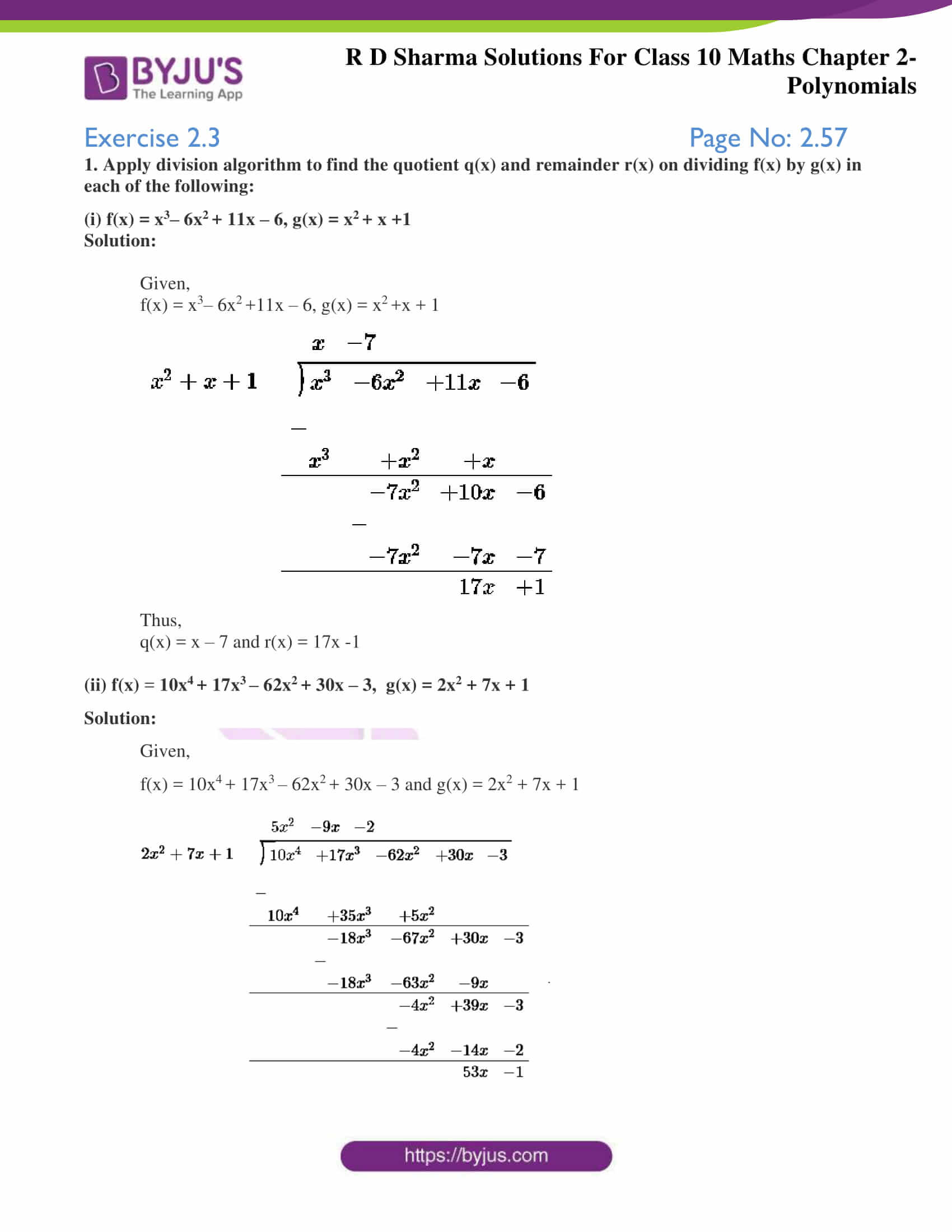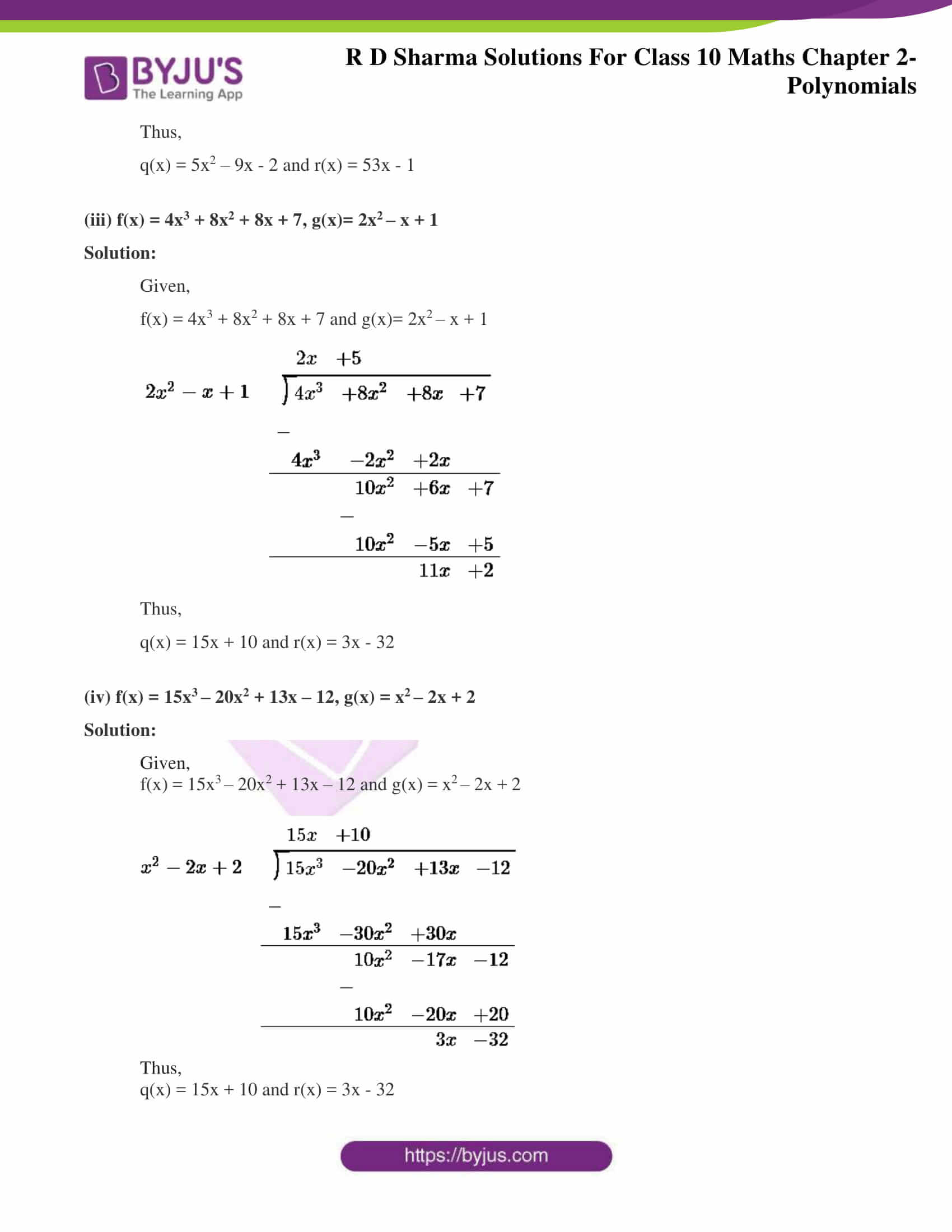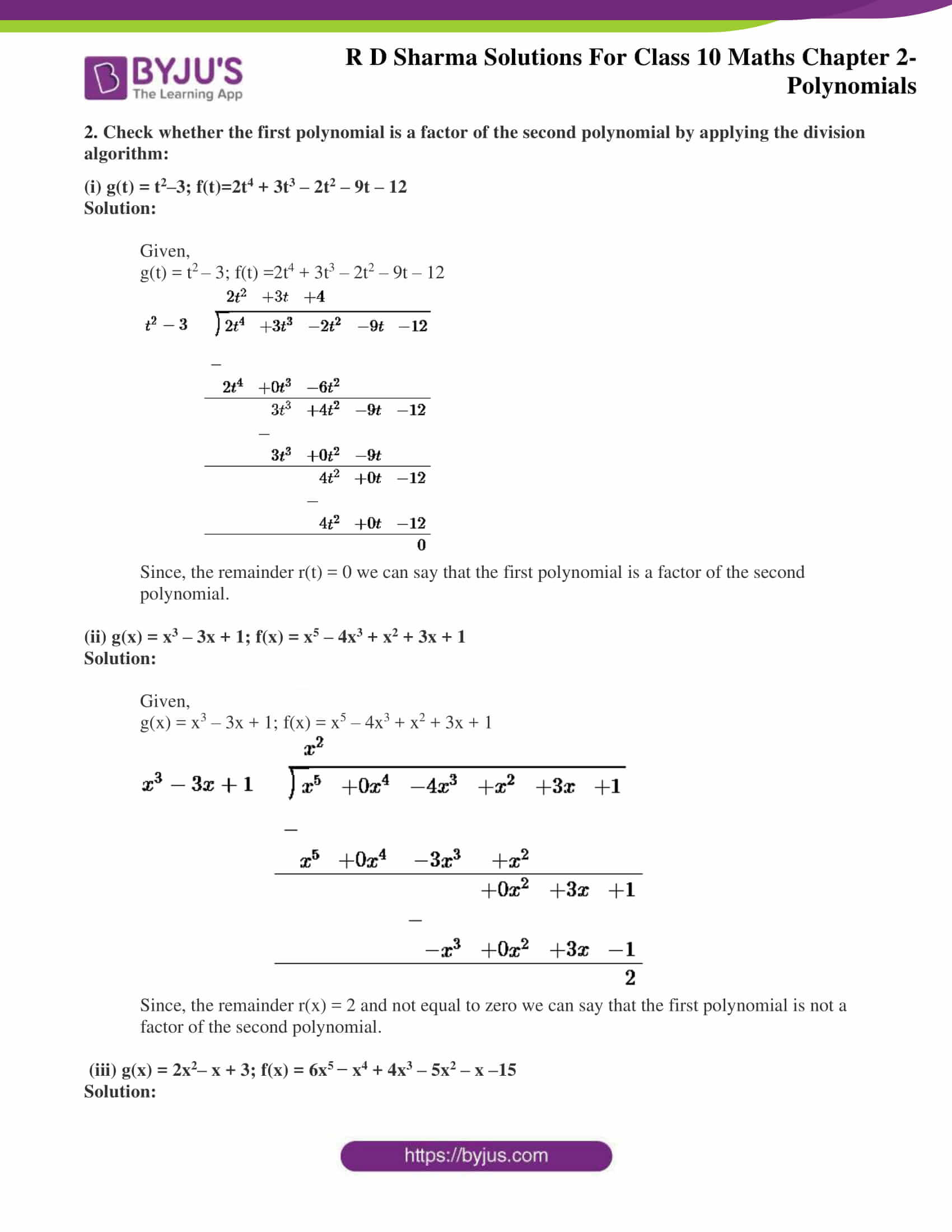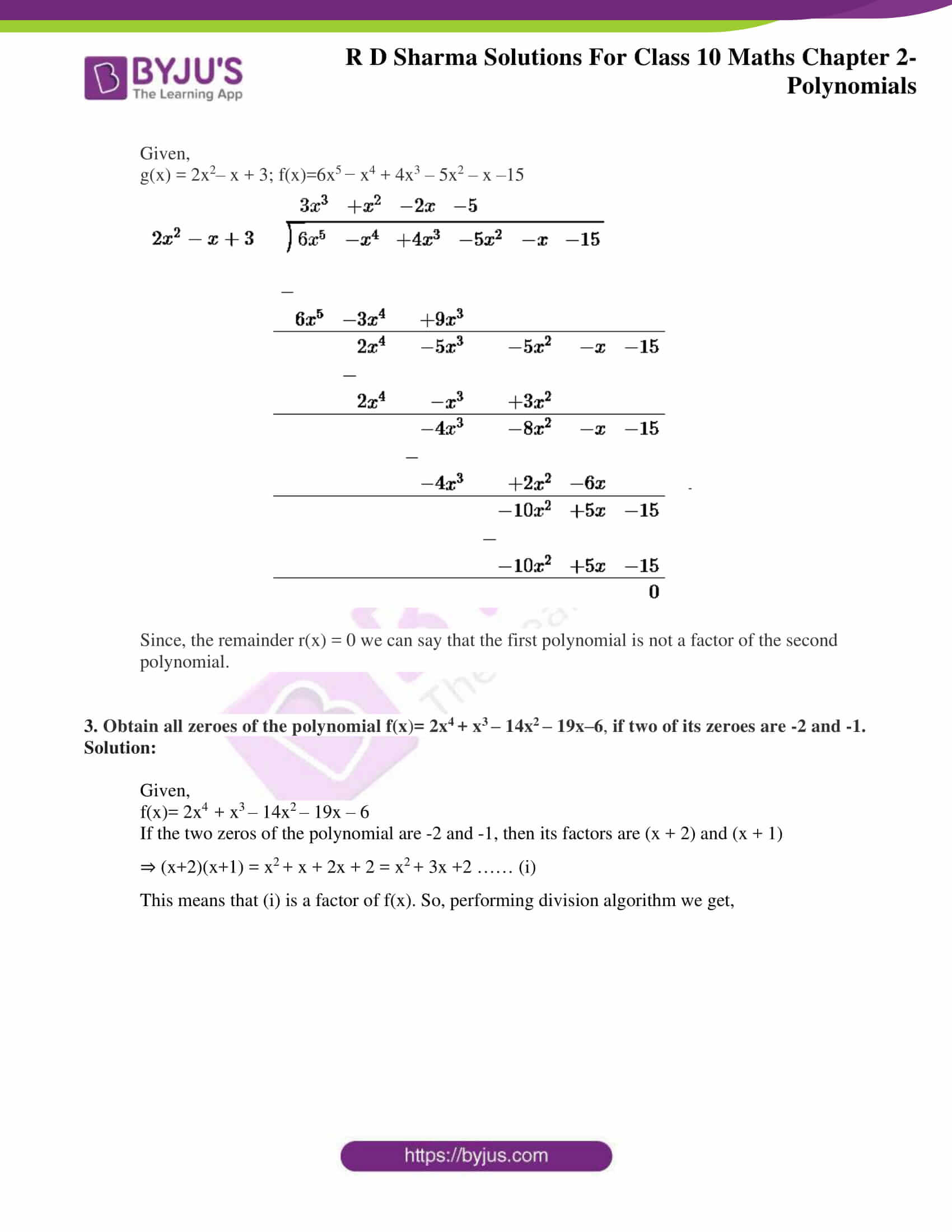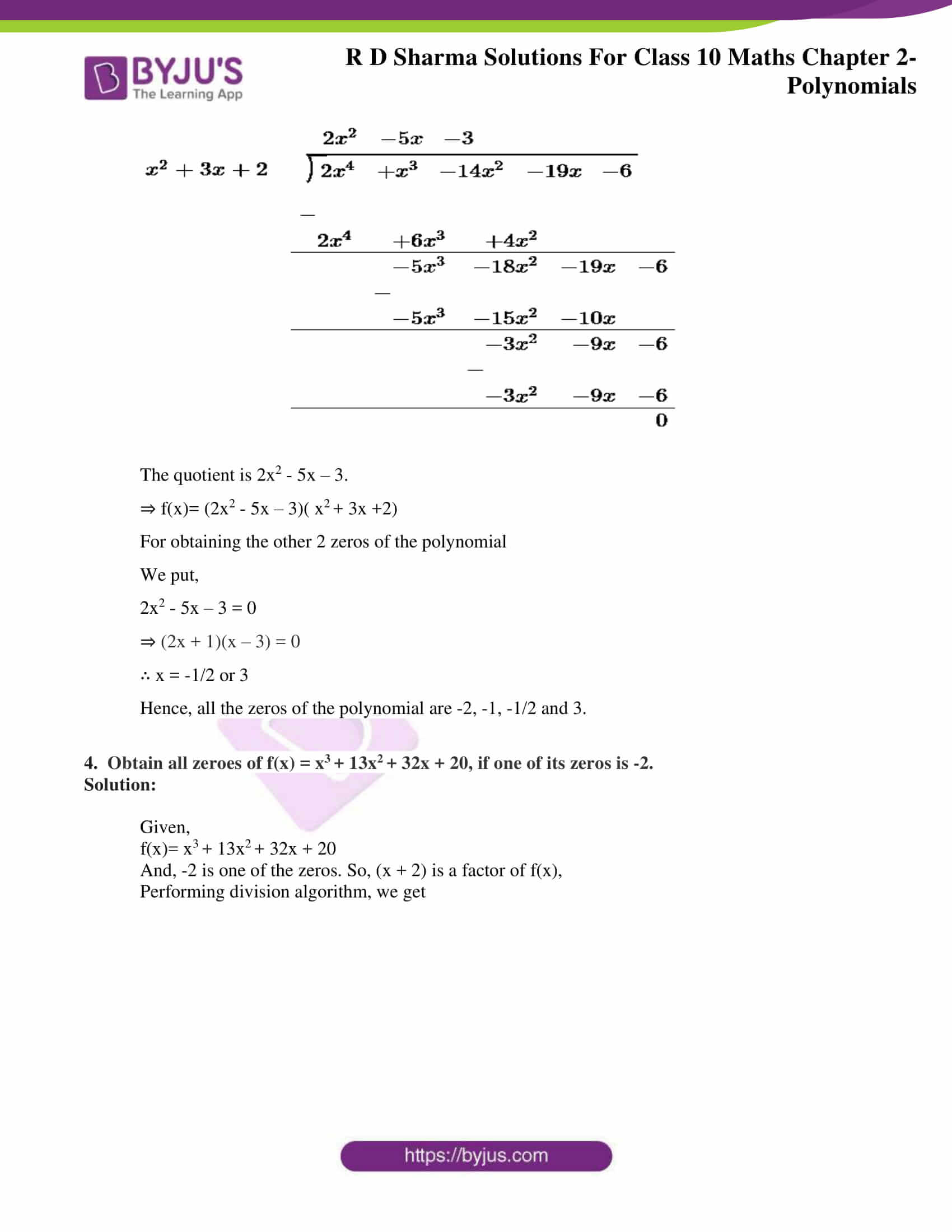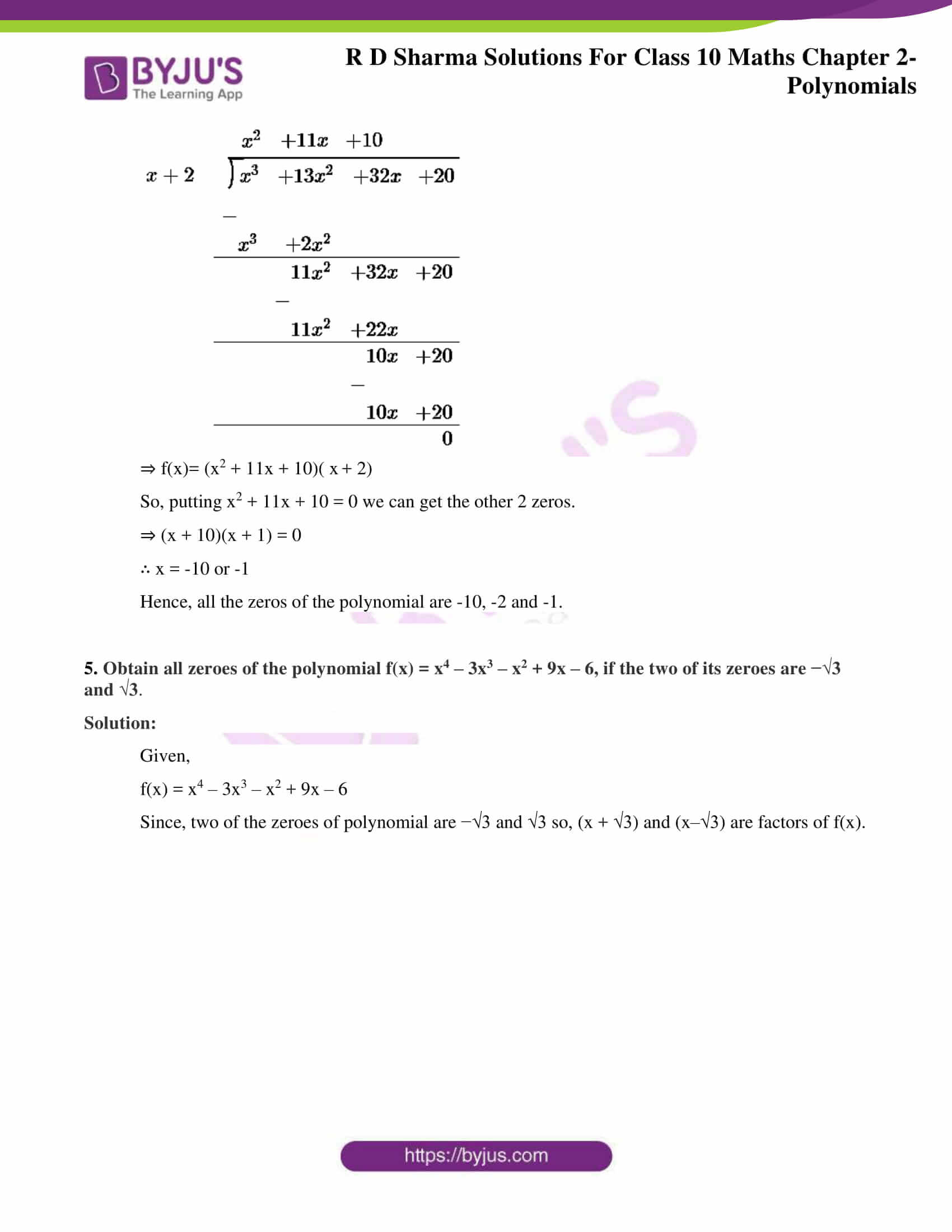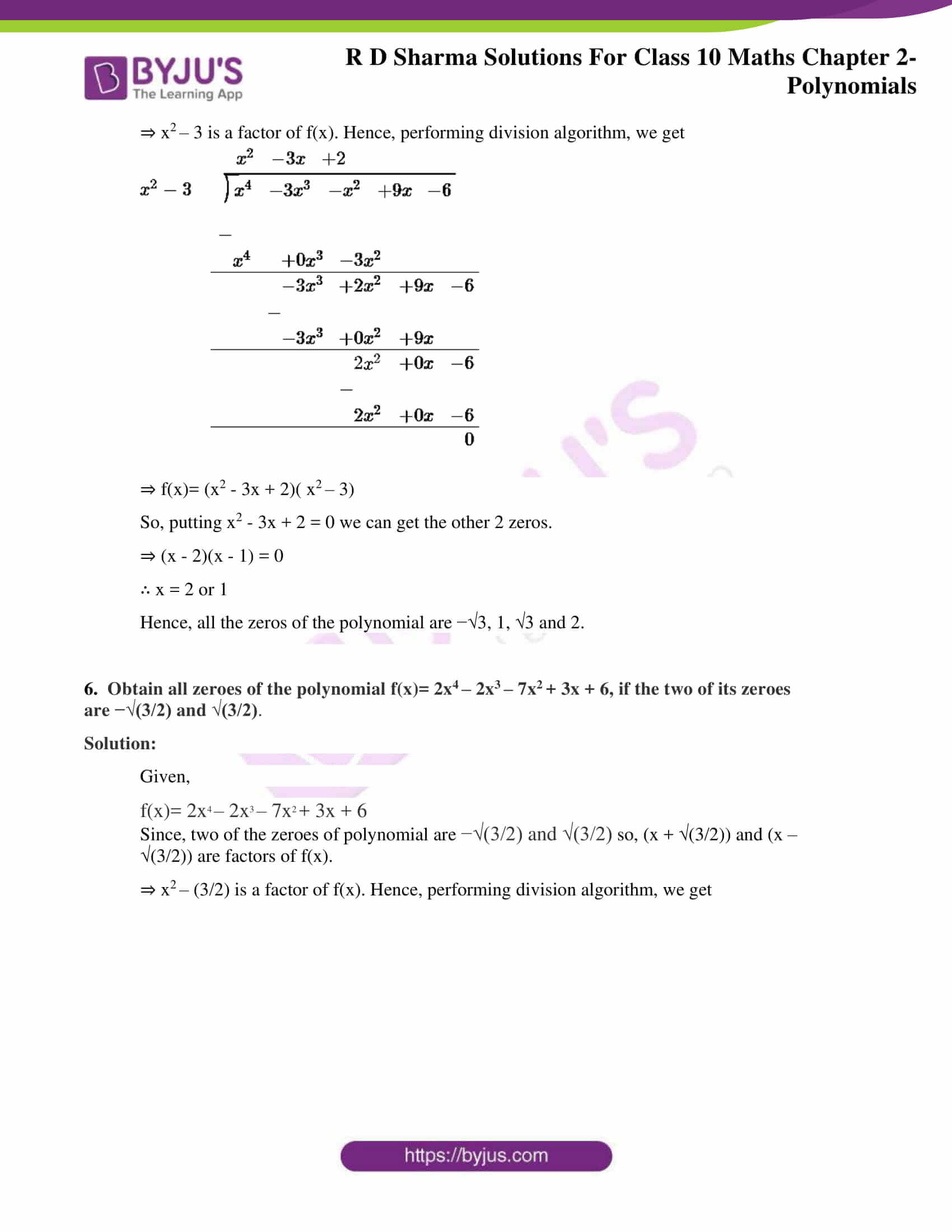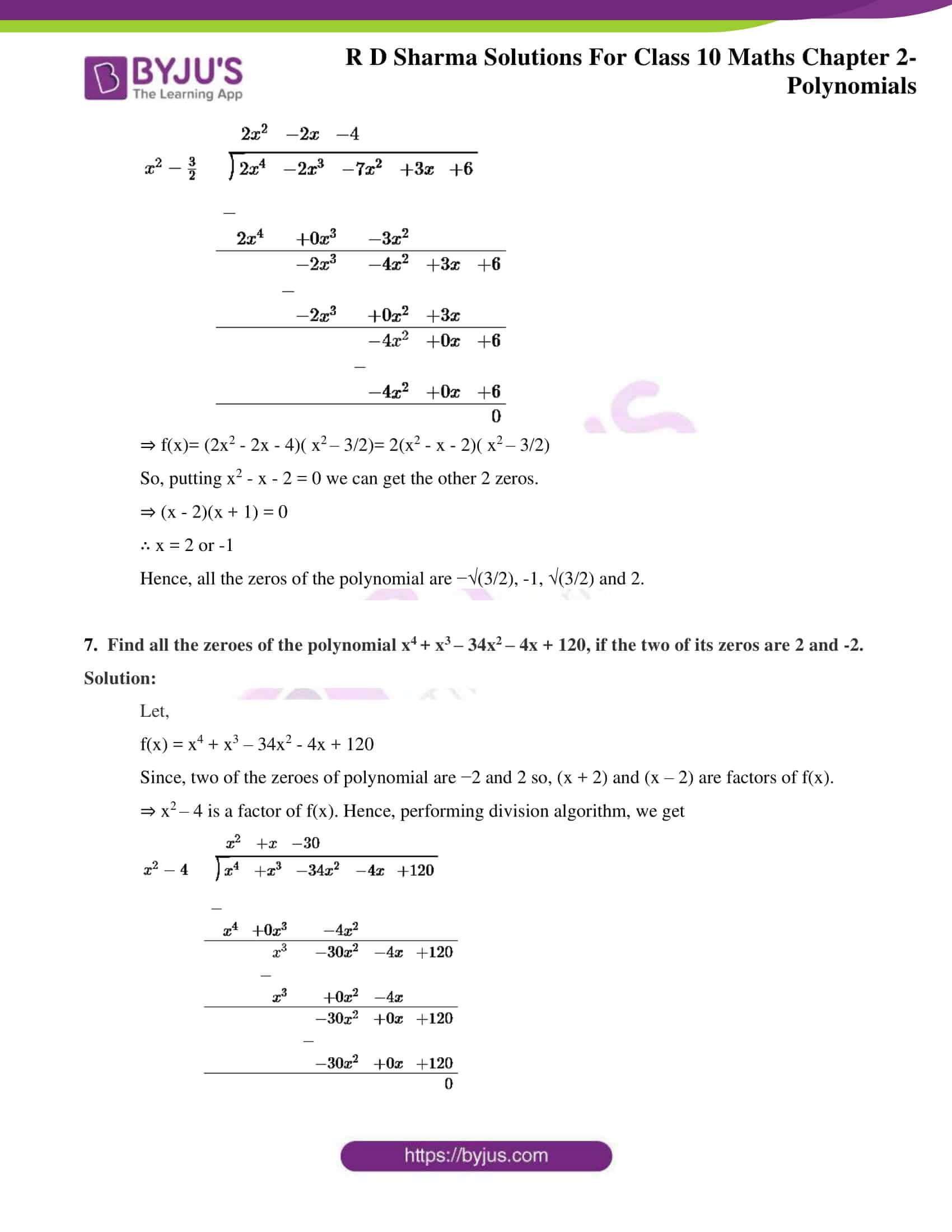### Access RD Sharma Solutions for Class 10 Chapter 2 Polynomials Exercise 2.3

1. Apply division algorithm to find the quotient q(x) and remainder r(x) on dividing f(x) by g(x) in each of the following:

(i) f(x) = x3– 6x2 + 11x – 6, g(x) = x2 + x +1

Solution:

Given,

f(x) = x3– 6x2 +11x – 6, g(x) = x2 +x + 1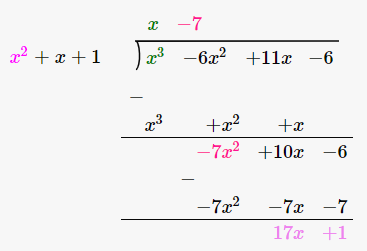Thus,

q(x) = x – 7 and r(x) = 17x -1

(ii) f(x) = 10x4 + 17x3 – 62x2 + 30x – 3, g(x) = 2x2 + 7x + 1

Solution:

Given,

f(x) = 10x4 + 17x3 – 62x2 + 30x – 3 and g(x) = 2x2 + 7x + 1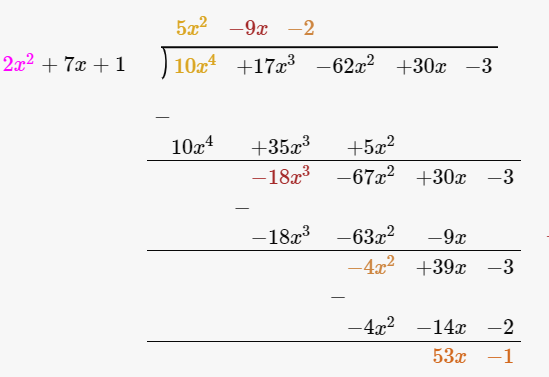Thus,

q(x) = 5x2 – 9x – 2 and r(x) = 53x – 1

(iii) f(x) = 4x3 + 8x2 + 8x + 7, g(x)= 2x2 – x + 1

Solution:

Given,

f(x) = 4x3 + 8x2 + 8x + 7 and g(x)= 2x2 – x + 1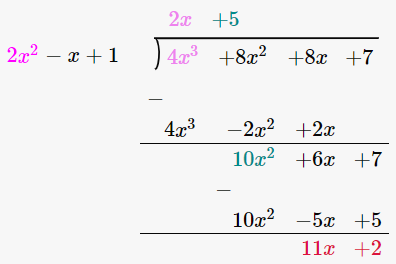Thus,

q(x) = 15x + 10 and r(x) = 3x – 32

(iv) f(x) = 15x3 – 20x2 + 13x – 12, g(x) = x2 – 2x + 2

Solution:

Given,

f(x) = 15x3 – 20x2 + 13x – 12 and g(x) = x2 – 2x + 2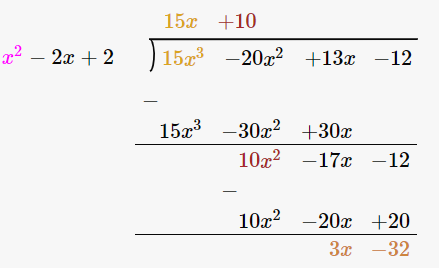Thus,

q(x) = 15x + 10 and r(x) = 3x – 32

2. Check whether the first polynomial is a factor of the second polynomial by applying the division algorithm:

(i) g(t) = t2–3; f(t)=2t4 + 3t3 – 2t2 – 9t – 12

Solution:

Given,

g(t) = t2 – 3; f(t) =2t4 + 3t3 – 2t2 – 9t – 12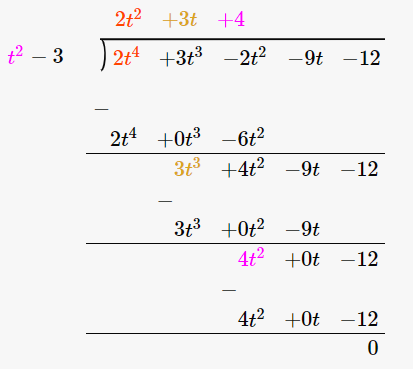Since, the remainder r(t) = 0 we can say that the first polynomial is a factor of the second polynomial.

(ii) g(x) = x3 – 3x + 1; f(x) = x5 – 4x3 + x2 + 3x + 1

Solution:

Given,

g(x) = x3 – 3x + 1; f(x) = x5 – 4x3 + x2 + 3x + 1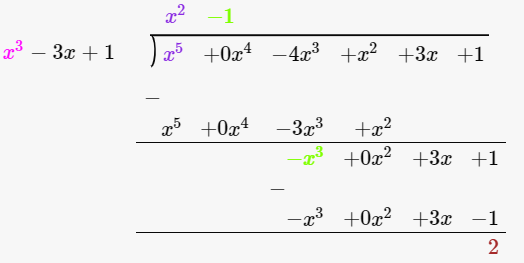Since, the remainder r(x) = 2 and not equal to zero we can say that the first polynomial is not a factor of the second polynomial.

(iii) g(x) = 2x2– x + 3; f(x) = 6x5 − x4 + 4x3 – 5x2 – x –15

Solution:

Given,

g(x) = 2x2– x + 3; f(x)=6x5 − x4 + 4x3 – 5x2 – x –15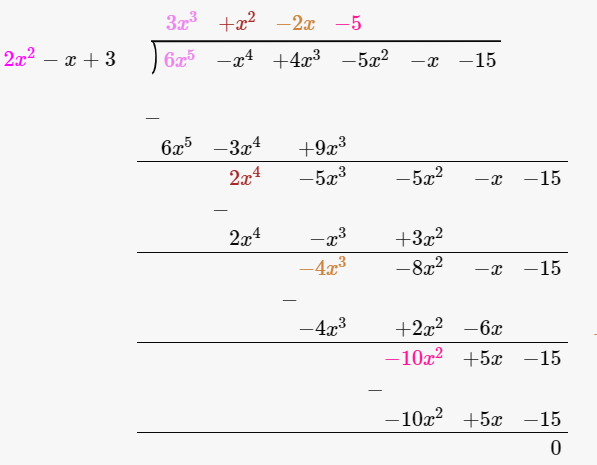Since, the remainder r(x) = 0 we can say that the first polynomial is not a factor of the second polynomial.

3. Obtain all zeroes of the polynomial f(x)= 2x4 + x3 – 14x2 – 19x–6, if two of its zeroes are -2 and -1.

Solution:

Given,

f(x)= 2x4 + x3 – 14x2 – 19x – 6

If the two zeros of the polynomial are -2 and -1, then its factors are (x + 2) and (x + 1)

⇒ (x+2)(x+1) = x2 + x + 2x + 2 = x2 + 3x +2 …… (i)

This means that (i) is a factor of f(x). So, performing division algorithm we get,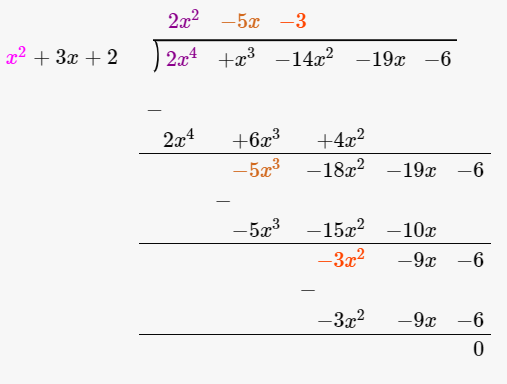The quotient is 2x2 – 5x – 3.

⇒ f(x)= (2x2 – 5x – 3)( x2 + 3x +2)

For obtaining the other 2 zeros of the polynomial

We put,

2x2 – 5x – 3 = 0

⇒ (2x + 1)(x – 3) = 0

∴ x = -1/2 or 3

Hence, all the zeros of the polynomial are -2, -1, -1/2 and 3.

4.  Obtain all zeroes of f(x) = x3 + 13x2 + 32x + 20, if one of its zeros is -2.

Solution:

Given,

f(x)= x3 + 13x2 + 32x + 20

And, -2 is one of the zeros. So, (x + 2) is a factor of f(x),

Performing division algorithm, we get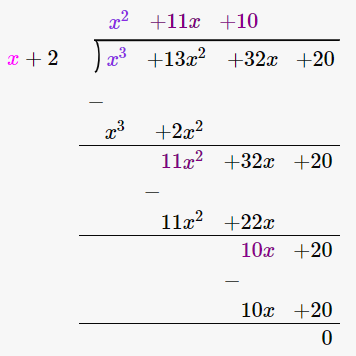⇒ f(x)= (x2 + 11x + 10)( x + 2)

So, putting x2 + 11x + 10 = 0 we can get the other 2 zeros.

⇒ (x + 10)(x + 1) = 0

∴ x = -10 or -1

Hence, all the zeros of the polynomial are -10, -2 and -1.

5. Obtain all zeroes of the polynomial f(x) = x4 – 3x3 – x2 + 9x – 6, if the two of its zeroes are −√3 and √3.

Solution:

Given,

f(x) = x4 – 3x3 – x2 + 9x – 6

Since, two of the zeroes of polynomial are −√3 and √3 so, (x + √3) and (x–√3) are factors of f(x).

⇒ x2 – 3 is a factor of f(x). Hence, performing division algorithm, we get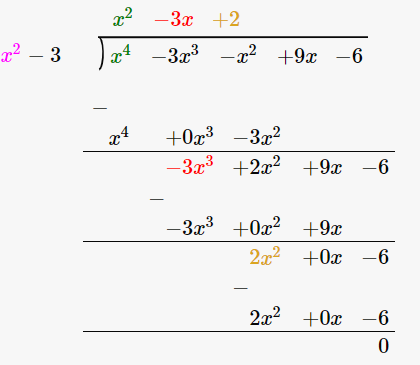⇒ f(x)= (x2 – 3x + 2)( x2 – 3)

So, putting x2 – 3x + 2 = 0 we can get the other 2 zeros.

⇒ (x – 2)(x – 1) = 0

∴ x = 2 or 1

Hence, all the zeros of the polynomial are −√3, 1, √3 and 2.

6.  Obtain all zeroes of the polynomial f(x)= 2x4 – 2x3 – 7x2 + 3x + 6, if the two of its zeroes are −√(3/2) and √(3/2).

Solution:

Given,

f(x)= 2x4 – 2x3 – 7x2 + 3x + 6

Since, two of the zeroes of polynomial are −√(3/2) and √(3/2) so, (x + √(3/2)) and (x –√(3/2)) are factors of f(x).

⇒ x2 – (3/2) is a factor of f(x). Hence, performing division algorithm, we get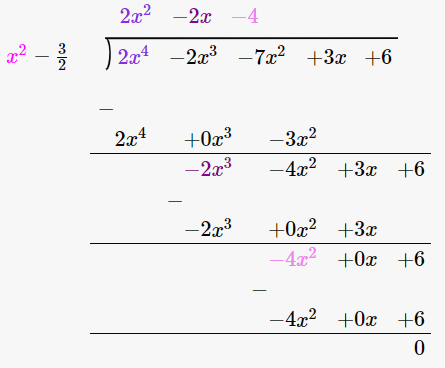⇒ f(x)= (2x2 – 2x – 4)( x2 – 3/2)= 2(x2 – x – 2)( x2 – 3/2)

So, putting x2 – x – 2 = 0 we can get the other 2 zeros.

⇒ (x – 2)(x + 1) = 0

∴ x = 2 or -1

Hence, all the zeros of the polynomial are −√(3/2), -1, √(3/2) and 2.

7.  Find all the zeroes of the polynomial x4 + x3 – 34x2 – 4x + 120, if the two of its zeros are 2 and -2.

Solution:

Let,

f(x) = x4 + x3 – 34x2 – 4x + 120

Since, two of the zeroes of polynomial are −2 and 2 so, (x + 2) and (x – 2) are factors of f(x).

⇒ x2 – 4 is a factor of f(x). Hence, performing division algorithm, we get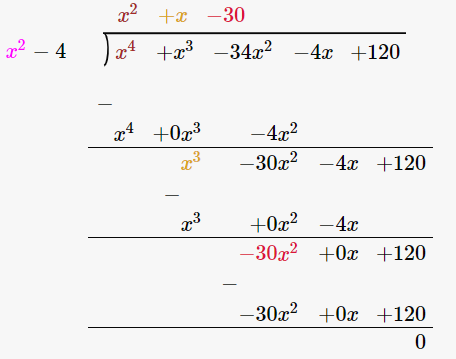⇒ f(x)= (x2 + x – 30)( x2 – 4)

So, putting x2 + x – 30 = 0 we can get the other 2 zeros.

⇒ (x – 6)(x + 5) = 0

∴ x = 6 or -5

Hence, all the zeros of the polynomial are -5, -2, 2 and 6.

### Also, access other exercise solutions of RD Sharma Class 10 Maths Chapter 2 Polynomials

Exercise 2.2 Solutions

#### 1 Comment

1. Ramanjot

Thanks for helping to solve sums